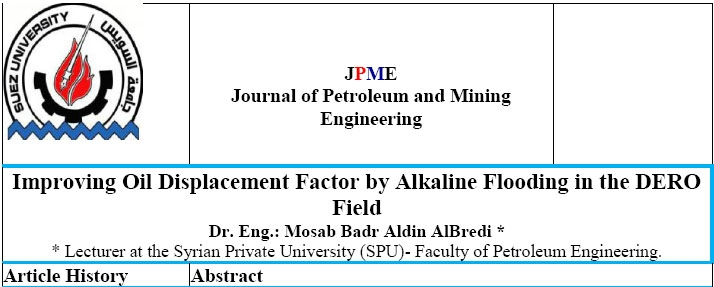### Improving Oil Displacement Factor by Alkaline Flooding in the DERO Field

Authors

Published in

JPME volume 18 (1) June 208

Abstract

In this research, a lab study was conducted on the process of injection a solution of Sodium Hydroxide (NaOH) into a lab-prepared a matrix Marble model that physically simulates the reservoir properties of the Jeribeh producing formation in DERO oil field. The Sodium Hydroxide (NaOH) solution is chosen due to its efficiency in studying alkaline. The injection operation of the alkaline was performed on the rock model using the volume ratios (15, 30 and 50% PV), (% PV that represents ratio of the injected liquid to the pores volume of the model), to guarantee an efficient displacement. The injection process was continued at each ratio using the displacement fluid until reaching the 250% PV level. Through the results of the displacement processes, the change of the following factors in relation with was graphically studied: where, Oil Displacement Factor (O.F.D), Water ratio in the produced fluid, (W) and: ratio of the produced oil volume at every injection process to the accumulated volume of the injected fluid at the same stage, (R.a=V_O/V_T ).
To compare the displacement efficiency using Sodium Hydroxide with the efficiency of water injecti

Attachement Files

Syrian Private University - Petrol Engineering @ 2023 by Syrian Monster - Web Service Provider | All Rights Reserved